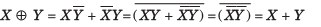Courses

# Number Systems, Boolean Algebra And Sequential Logic Circuits - MCQ Test

## 20 Questions MCQ Test RRB JE for Electronics & Communication Engineering | Number Systems, Boolean Algebra And Sequential Logic Circuits - MCQ Test

Description
This mock test of Number Systems, Boolean Algebra And Sequential Logic Circuits - MCQ Test for Railways helps you for every Railways entrance exam. This contains 20 Multiple Choice Questions for Railways Number Systems, Boolean Algebra And Sequential Logic Circuits - MCQ Test (mcq) to study with solutions a complete question bank. The solved questions answers in this Number Systems, Boolean Algebra And Sequential Logic Circuits - MCQ Test quiz give you a good mix of easy questions and tough questions. Railways students definitely take this Number Systems, Boolean Algebra And Sequential Logic Circuits - MCQ Test exercise for a better result in the exam. You can find other Number Systems, Boolean Algebra And Sequential Logic Circuits - MCQ Test extra questions, long questions & short questions for Railways on EduRev as well by searching above.
QUESTION: 1

Solution:
QUESTION: 2

### If (211)x = (152)8 , then the value of base x is

Solution: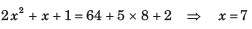QUESTION: 3

### 11001, 1001 and 111001 correspond to the 2’s complement representation of the following set of numbers

Solution:

All are 2’s complement of 7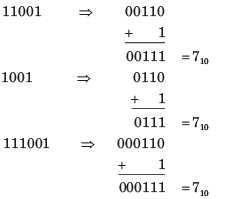QUESTION: 4

A signed integer has been stored in a byte using 2’s complement format. We wish to store the same integer in 16-bit word. We should copy the original byte to the less significant byte of the word and fill the more significant byte with

Solution:

See a example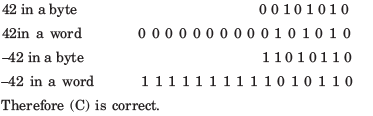QUESTION: 5

A computer has the following negative numbers stored in binary form as shown. The wrongly stored number is

Solution:
QUESTION: 6

The circuit shown in fig. is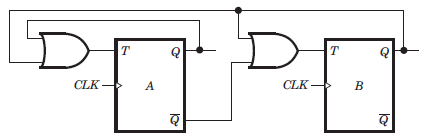Solution: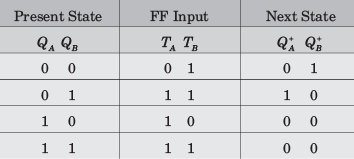From table it is clear that it is a MOD–3 counter.

QUESTION: 7

The counter shown in fig. is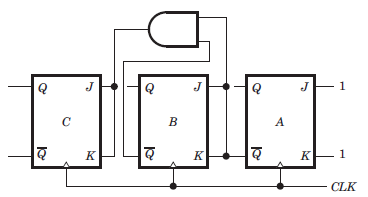Solution:

It is a down counter because 0 state of previous FFs change the state of next FF. You may trace the following sequence, let initial state be000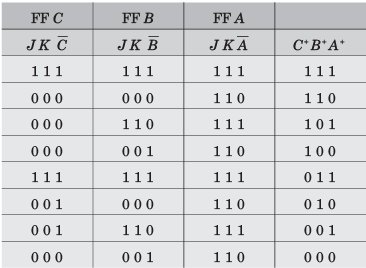QUESTION: 8

The counter shown in fig. counts from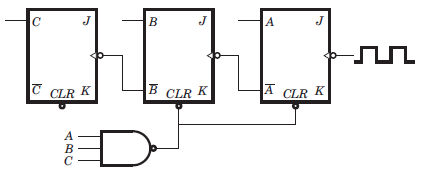Solution:

It is a down counter because the inverted FF output drive the clock inputs. The NAND gate will clear FFs A and B when the count tries to recycle to 111. This will produce as result of 100. Thus the counting sequence will be 100, 011, 010, 001, 000, 100 etc.

QUESTION: 9

The mod-number of the asynchronous counter shown in fig. is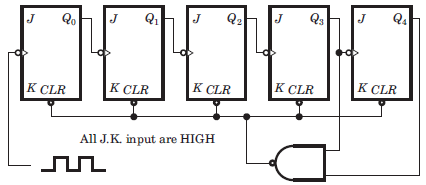Solution:

It is a 5 bit ripple counter. At 11000 the output of NAND gate is LOW. This will clear all FF. So it is a Mod 24 counter. Note that when 11000 occur, the CLR input is activated and all FF are immediately cleared. So it is a MOD 24 counter not MOD 25.

QUESTION: 10

The frequency of the pulse at z in the network shown in fig. is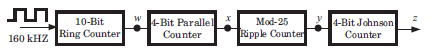Solution:

10-bit ring counter is a MOD–10, so it divides the 160 kHz input by 10. therefore, w = 16 kHz. The four-bit parallel counter is a MOD–16. Thus, the frequency at x = 1 kHz. The MOD–25 ripple counter produces a frequency at y = 40 Hz. (1 kHz/25 = 40 Hz).
The four-bit Johnson Counter is a MOD-8. This, the frequency at z = 5 Hz.

QUESTION: 11

The three-stage Johnson counter as shown in fig. is clocked at a constant frequency of fc from the starting state of Q2 Q1Q0 = 101. The frequency of output Q2 Q1Q0 will be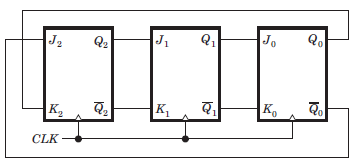Solution: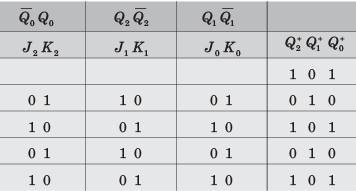We see that 1 0 1 repeat after every two cycles, hence frequency will be fc/2

QUESTION: 12

The counter shown in the fig. has initially Q2Q1Q0 = 000. The status of Q2 Q1Q after the first pulse is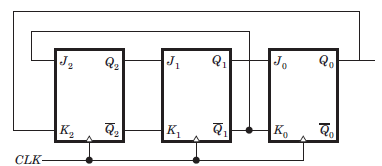Solution:

At first cycle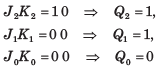QUESTION: 13

A 4 bit ripple counter and a 4 bit synchronous counter are made by flips flops having a propagation delay of 10 ns each. If the worst case delay in the ripple counter and the synchronous counter be R and S respectively, then

Solution:

In ripple counter delay 4Td = 40 ns.

The synchronous counter are clocked simultaneously, then its worst delay will be equal to 10 ns.

QUESTION: 14

A 4 bit modulo–6 ripple counter uses JK flip-flop. If the propagation delay of each FF is 50 ns, the maximum clock frequency that can be used is equal to

Solution:

4 bit uses 4 FF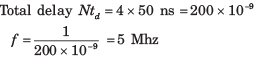QUESTION: 15

The initial contents of the 4-bit serial-in-parallel-out right-shift, register shown in fig. is 0 1 1 0. After three clock pulses are applied, the contents of the shift register will be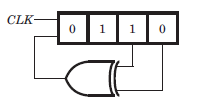Solution: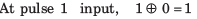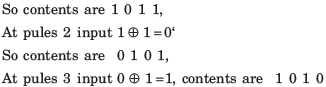QUESTION: 16

Consider the signed binary number A = 01010110 and B = 1110 1100 where B is the 1’s complement and MSB is the sign bit. In list-I operation is given, and in list-II resultant binary number is given.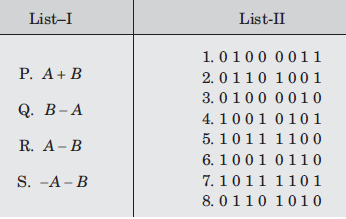The correct match is

Solution: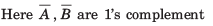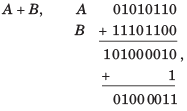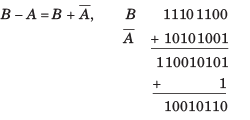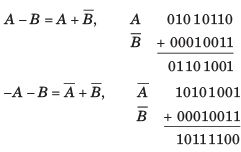QUESTION: 17

The simplified form of a logic function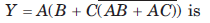Solution: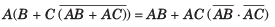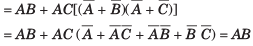QUESTION: 18

If the decimal number is a fraction then its binary equivalent is obtained by ________ the number continuously by 2.

Solution:

On multiplying the decimal number continuously by 2, the binary equivalent is obtained.

QUESTION: 19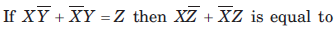Solution: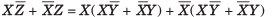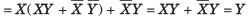QUESTION: 20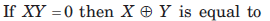Solution: